Can you filter your table data using an Excel FILTER Formula?

Yes, you can!

It is definitely possible now with Excel FILTER Formula. It is a new formula introduced in Office 365 released in 2018!

In this article, you will be provided with a detailed guide on:

### Excel Filter Formula

The FILTER formula in Excel is available in Office 365 only.

It is an in-built formula in Excel used to filter an array or range based on the criteria you specify and extract matching records.

It is part of Dynamic Array functions and the result provided by this formula is an array that spills on the worksheet automatically.

What does it do?

Filters a table array based on the filtering condition given

Formula breakdown:

=FILTER(array, include, [if_empty])

What it means:

• array – data to be filtered.
• include – logical test supplied as criteria. It should have the same height or width as the array.
• if_empty – value to display if the filter results in no records. It is an optimal argument.

Generally, Excel user filters data using the Autofilter button present under the Data Tab. But the drawback of using the filter buttons is that it does not update results automatically when you make changes in the data.

Whereas, the Excel FILTER function in Excel is dynamic i.e. when the values in the data sources change the result from this formula will update automatically.

### Want to know How To Master the Excel FILTER Formula?

Watch on YouTube and give it a thumbs up 👍In the example below, we have a tax table that we want to dynamically filter with a given rate. So, we want to extract the income levels for which the tax rate is greater than 33%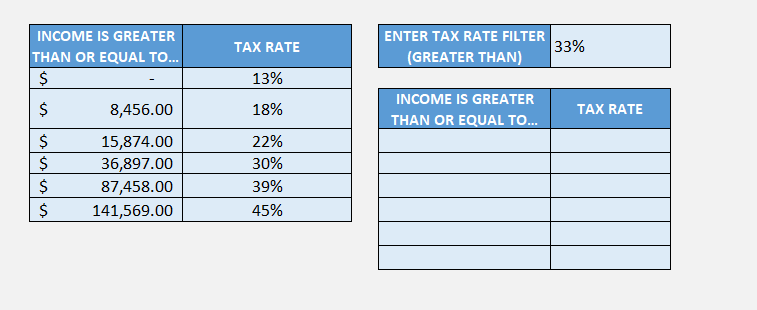STEP 1: We need to enter the FILTER function in a blank cell:

## =FILTER(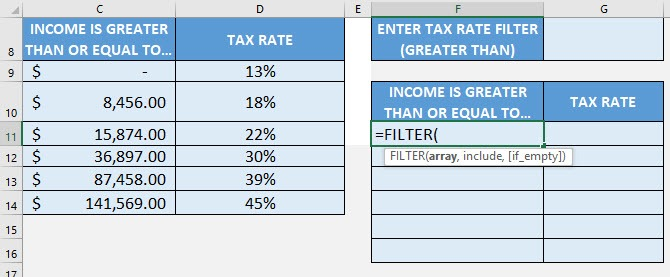STEP 2: The Excel FILTER formula arguments:

## array

What is the data to be filtered?

Select the cells containing the tax data, do not include the headers:

## =FILTER(C9:D14,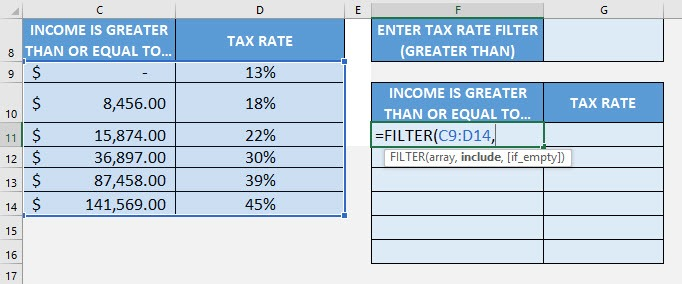## include

We want to filter the tax rate that is greater than the specified rate. Type in the condition as the tax rate column > the specific tax rate.

## =FILTER(C9:D14, D9:D14>G8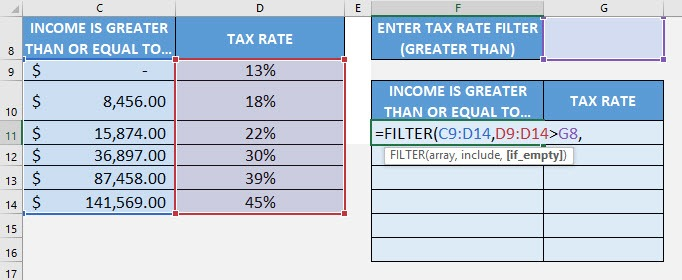## [if_empty]

What is the value to display in case nothing gets matched?

Just place an empty string to be displayed if nothing gets matched.

## =FILTER(C9:D14, D9:D14>G8, “”)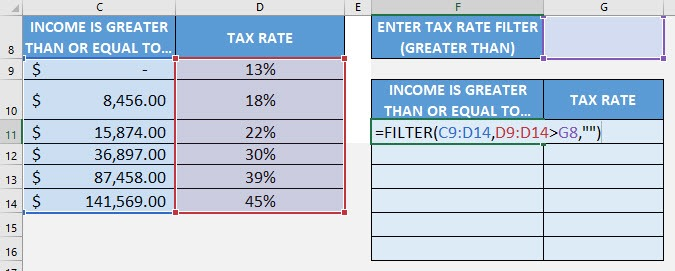Try it out now with different values and see it get filtered magically!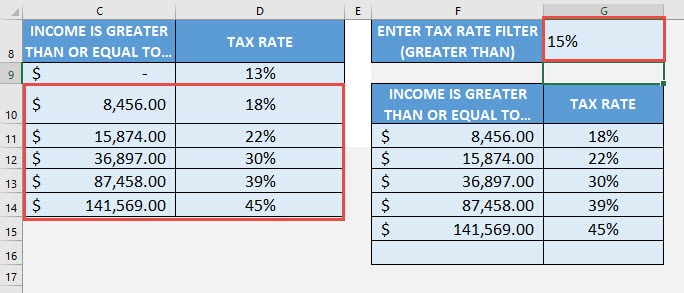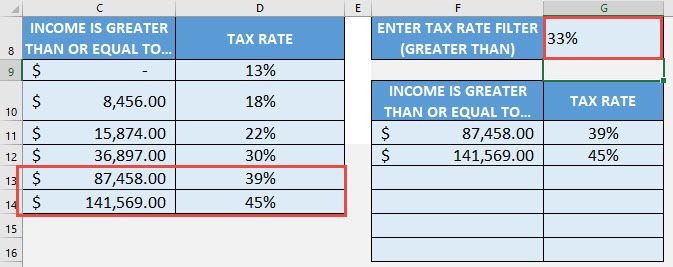This formula can handle multiple criteria as well. Let’s check it out!

### Filtering with Multiple Criteria

In this example, we have sales data and we want to filter data when the salesperson is Ian Wright and Product is Tonic.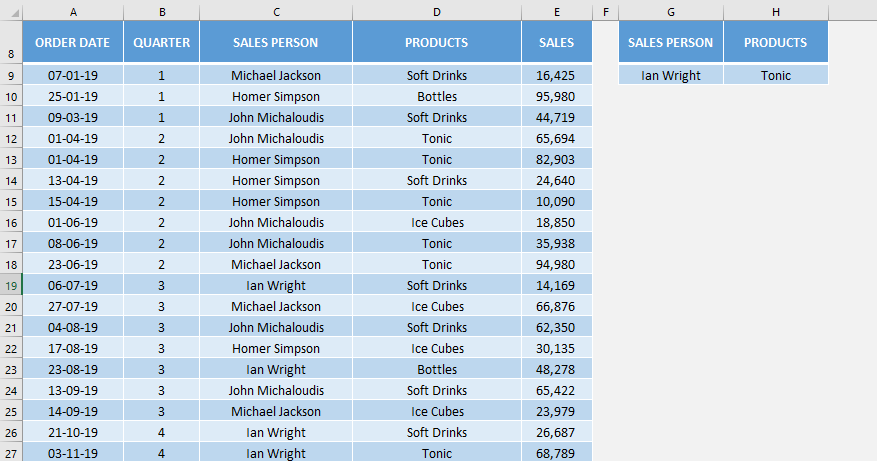Let’s use the FILTER formula wth multiple criteria and get this done!

STEP 1: Enter the FILTER function in cell G12.

## =FILTER(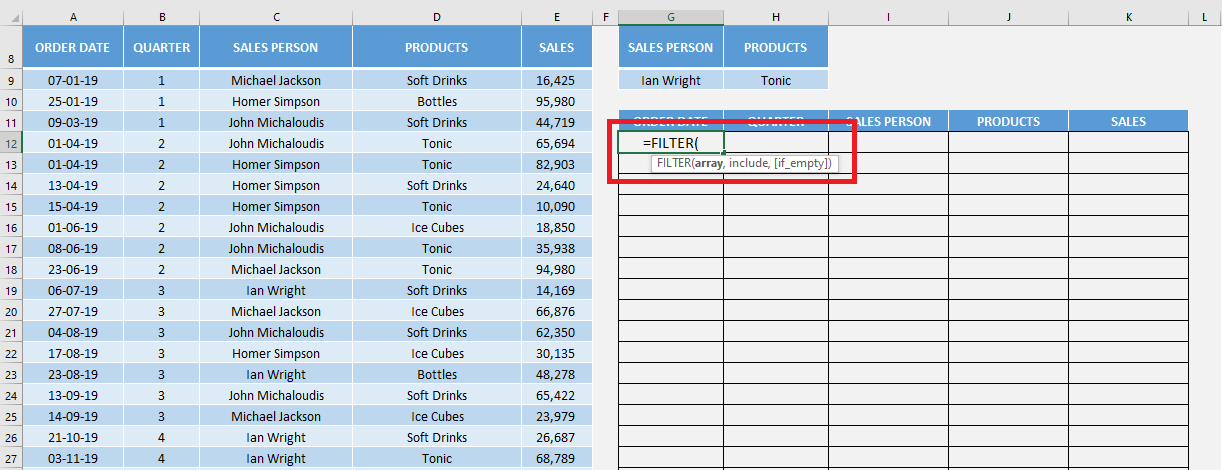STEP 2: Select the array that needs to be filtered i.e. A9: E56

## =FILTER(A9:E56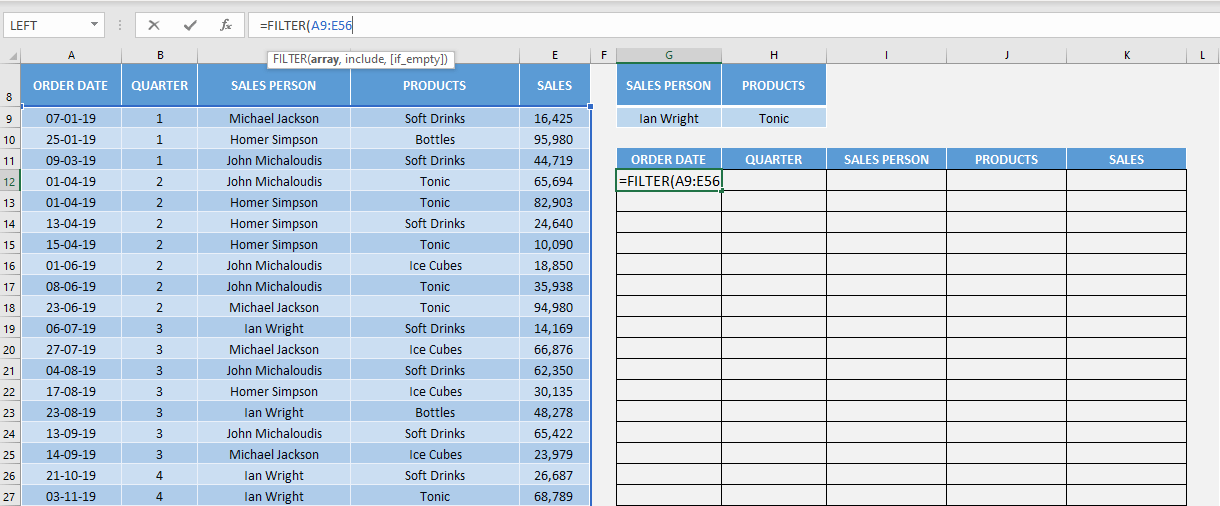Now, you add multiple criteria you need to make sure that the two logical tests are separated by a multiplication operator.

=FILTER(array, (range1=criteria1) * (range2=criteria2))

STEP 2: Add the first range (salesperson) and its criteria (Ian Wright) i.e. C9: C56 and G9 respectively.

## =FILTER(A9:E56, (C9:C56=G9)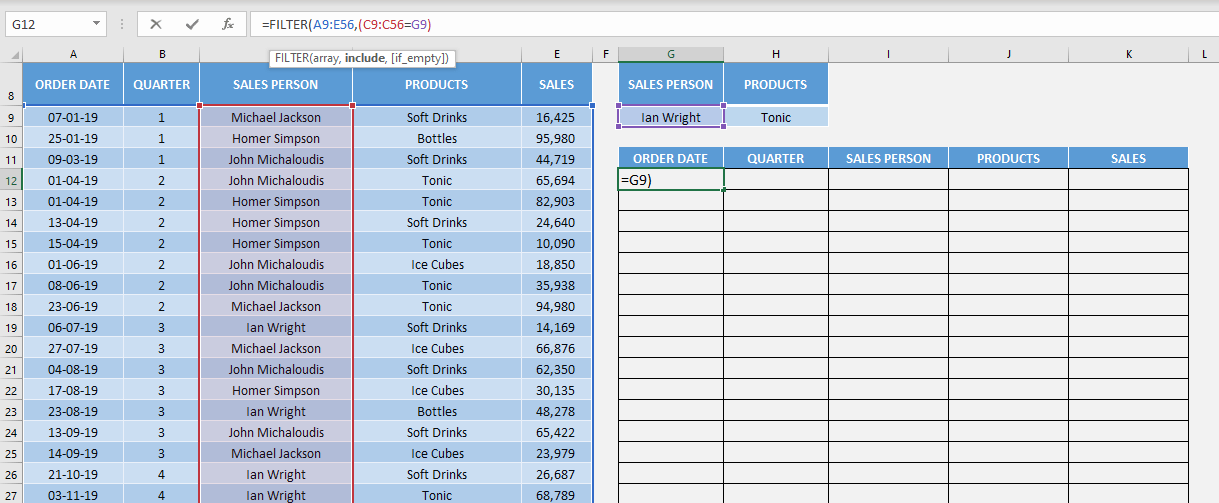STEP 3: Insert a Multiplication Operator and then add the second range (product) and its criteria (Tonic) i.e. D9: D56 and H9 respectively.

## =FILTER(A9:E56, (C9:C56=G9) *(D9:D56=H9)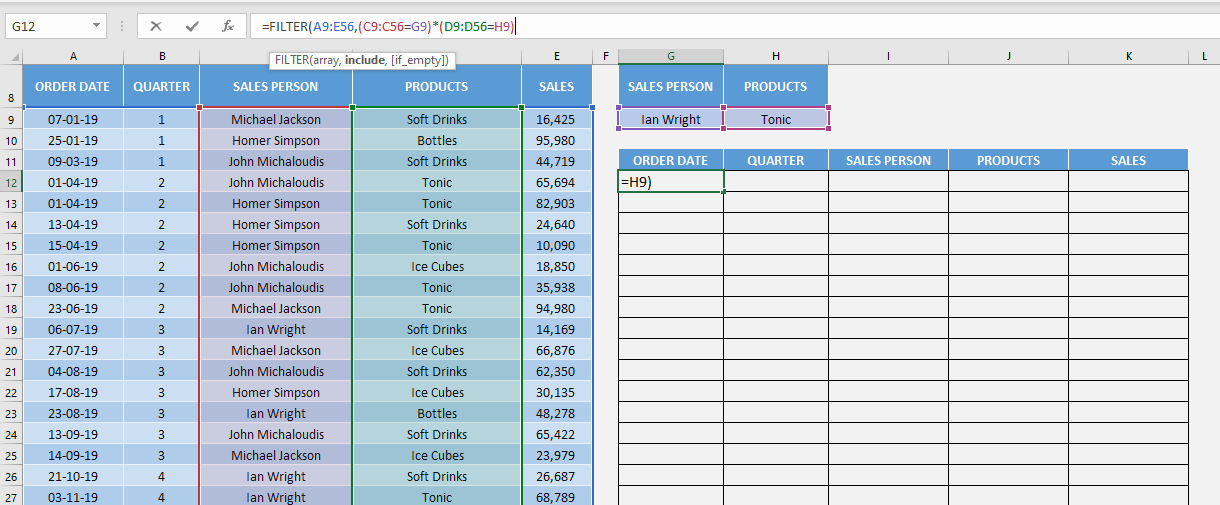STEP 4: Add the text to display if the filtering results in no record i.e. No Entry Found.

## =FILTER(A9:E56, (C9:C56=G9) *(D9:D56=H9),”No Entry Found”)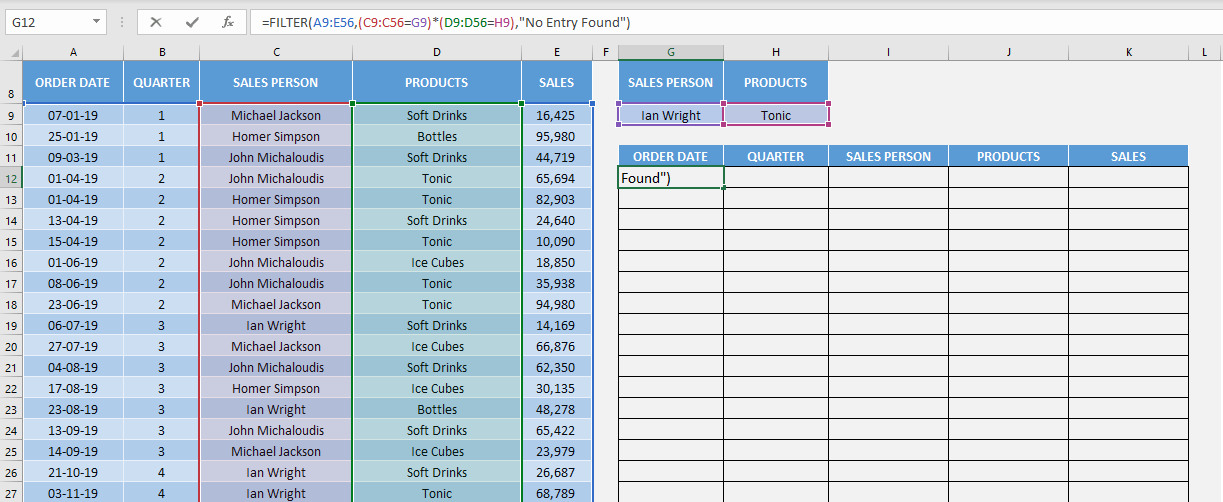This is how you can use the Excel FILTER formula with single or multiple criteria.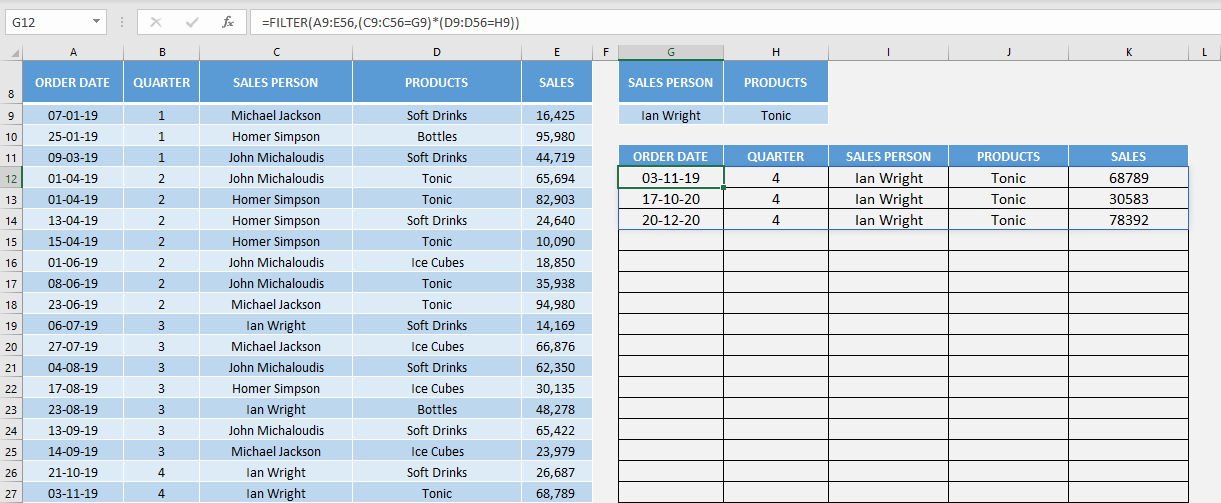Byt, there may be times when you might encounter an error when using filter function Excel. Let’s look at some of those errors and how to correct them!

### Filter formula not working

#SPILL Error

This error occurs if one or more cells where the result will be displayed in not blank.

In this example, as you can see there is already text (-) in cell G13. The FILTER formula is showing error.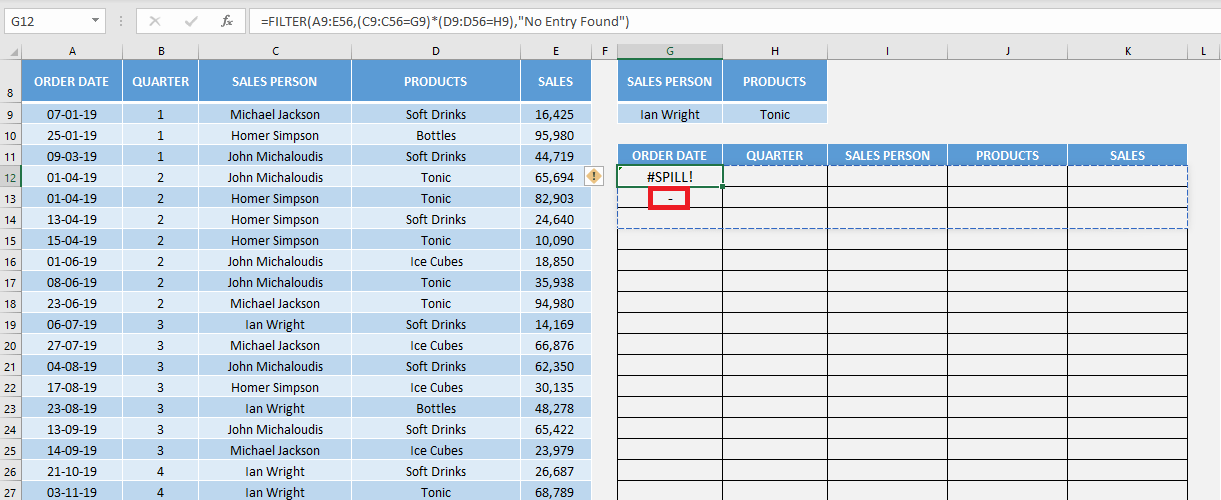To fix it, simply delete any contents present in those cells and the desired result will appear.#CALC! Error

This error occurs when if_empty is omitted and there is no entry matching the criteria provided.

In this example, as you can see there is no text present of if_empty argument and there are no records for salesperson Ian Wright and product Drinks, there is an #CALC! error.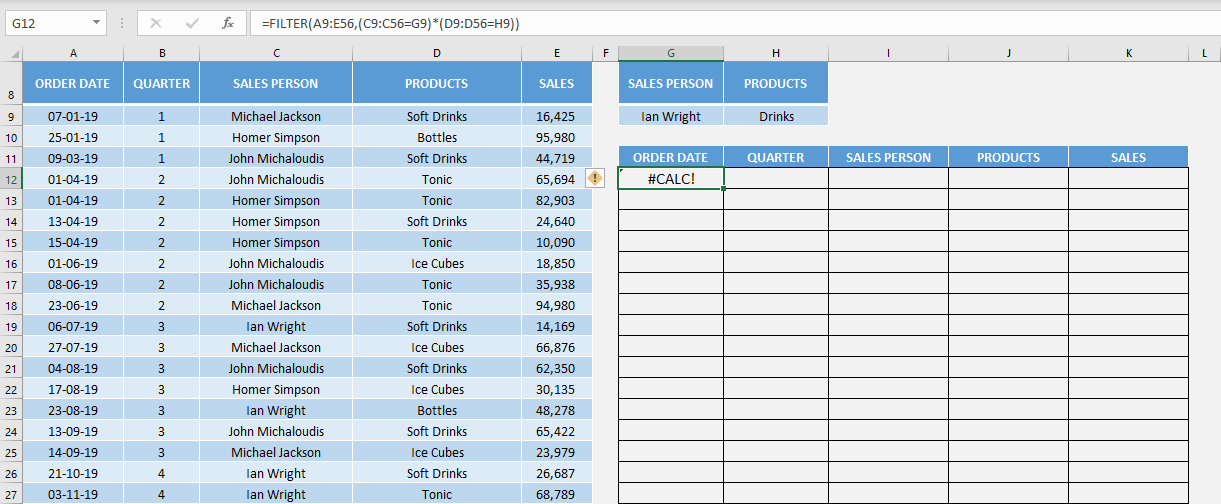To fix it, simply add a text in the formula’s if_empty argument.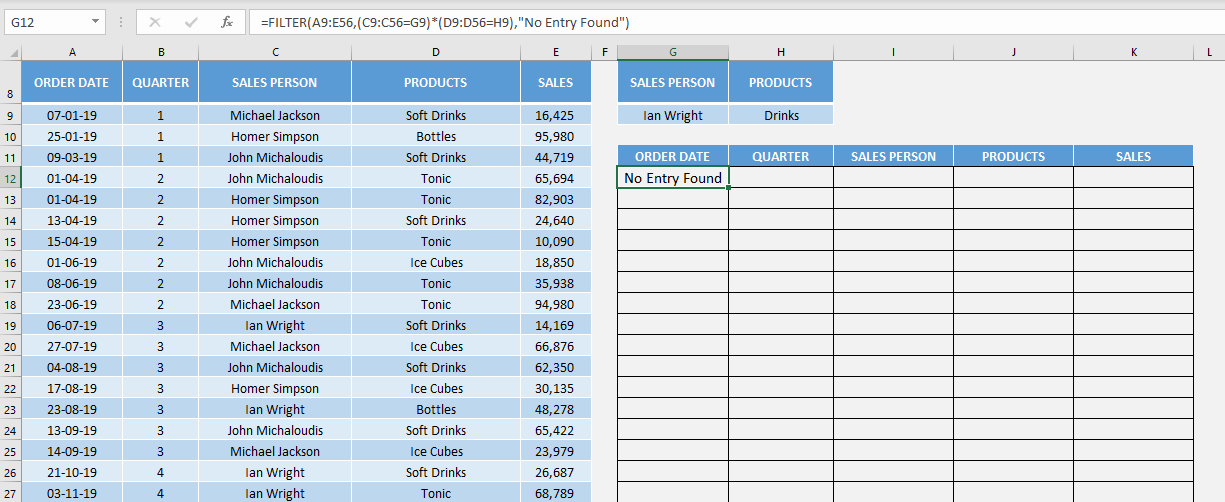#VALUE Error

One of the reasons why no may encounter this error is that neither the height or width of the include argument matches that of the array argument.

In this example, the height of the array is from row 9 to row 56 but height for include is for row 9 to row 50. So, Excel displays an #VALUE! error!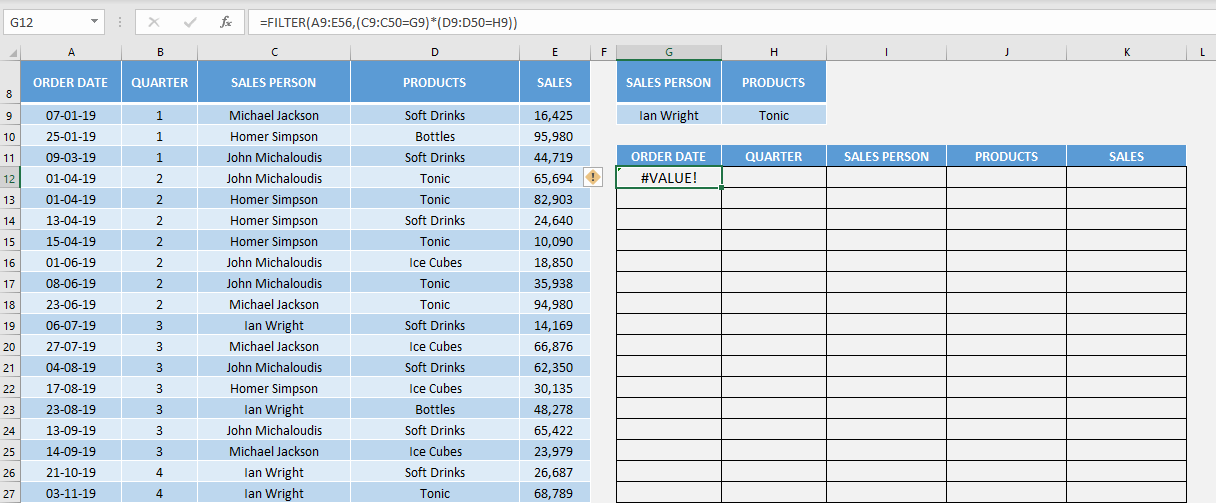To fix it, simply make sure that the height or width of include is the same as the array.